## Nitrogen:

There are two methods for estimation of nitrogen: (i) Dumas method and (ii) Kjeldahl’s method.

### Dumas Method:

A known mass of organic compound is heated with excess of CuO in an atmosphere of CO2, when nitrogen of the organic compound is converted into N2 gas.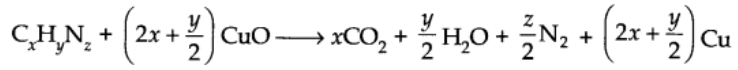The volume of N2 thus obtained is converted into STP and the percentage of nitrogen determined by applying the equation: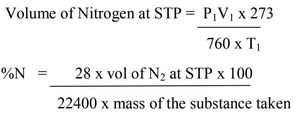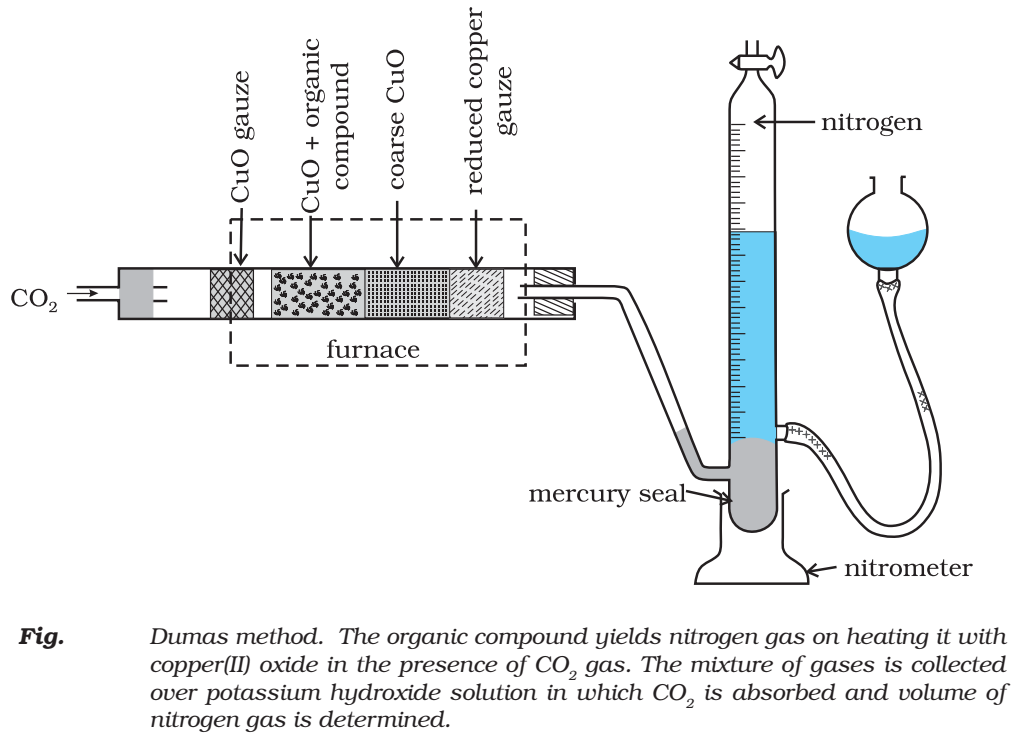### Kjeldahl’s Methods:

Kjeldahl’s method is based on the fact that when an organic compound containing nitrogen is heated with con. H2S04 the nitrogen in it is converted to ammonium sulphate. The resultant liquid is then treated with excess of alkali and the liberated ammonia gas absorbed in excess of standard acid. The amount of ammonia is determined by finding the amount of acid neutralised by back filtration with some std. alkali.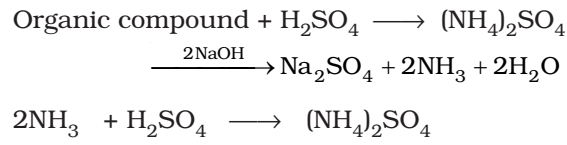Volume of acid of normality N1 neutralised by NH3 = V1 cm3

1000 cm3 of 1N NH3 = 17 g of NH3

V1 cm3 of N1 acid = 14 g of Nitrogen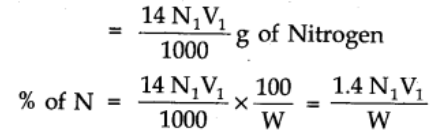where N1 is the normality of acid.

V1 = volume of acid.

W = weight of organic compound.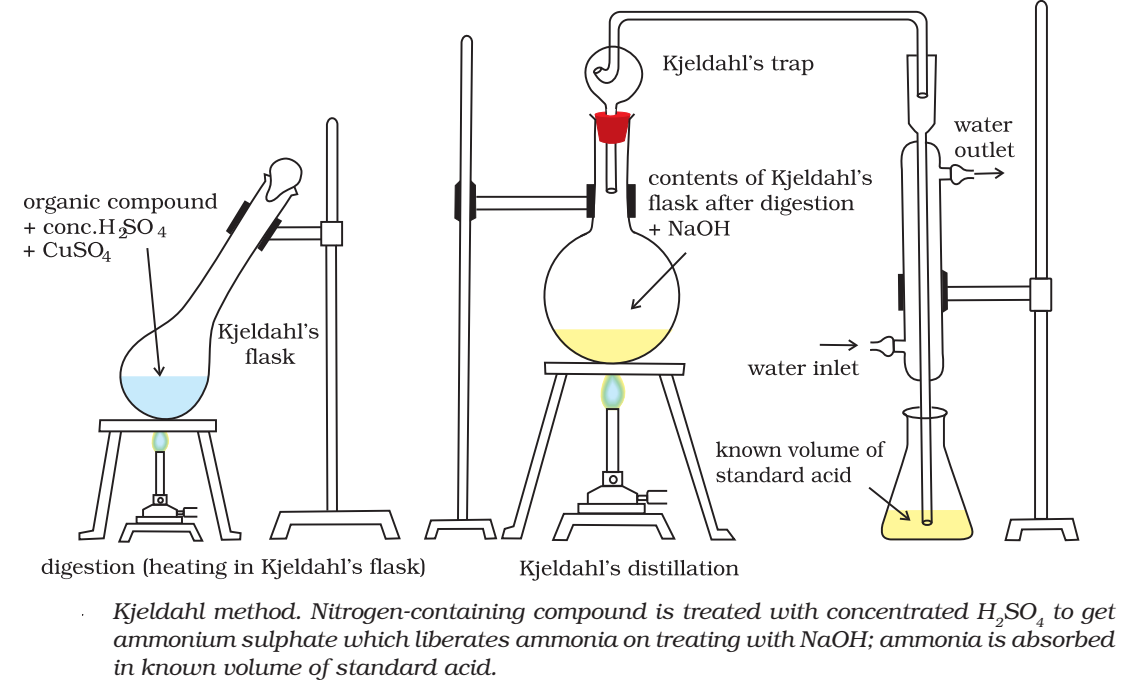## Halogens

### Carius method:

A known mass of an organic compound is heated with fuming nitric acid in the presence of silver nitrate contained in a hard glass tube known as Carius tube, (Fig.) in a furnace. Carbon and hydrogen present in the compound are oxidised to carbon dioxide and water. The halogen present forms the corresponding silver halide (AgX). It is filtered, washed, dried and weighed.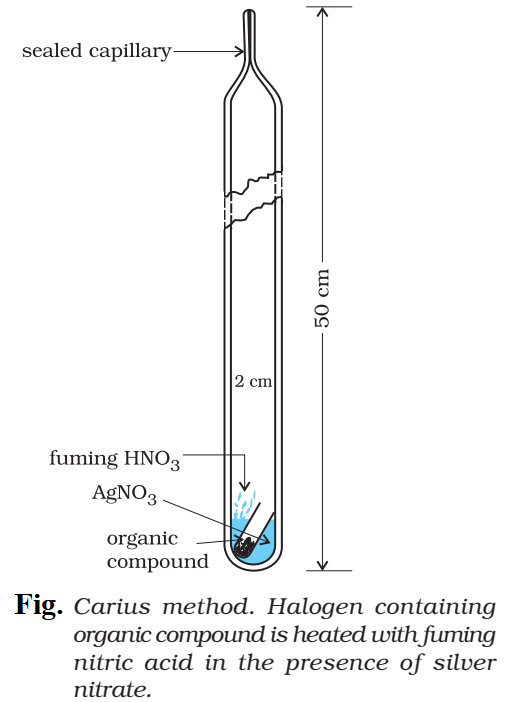Let the mass of the organic compound taken = m g

Mass of AgX formed = m1 g

1 mol of AgX contains 1 mol of X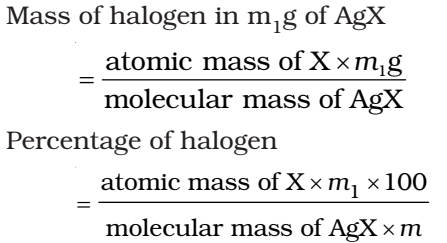## Sulphur:

A known mass of an organic compound is heated in a Carius tube with sodium peroxide or fuming nitric acid. Sulphur present in the compound is oxidised to sulphuric acid. It is precipitated as barium sulphate by adding excess of barium chloride solution in water.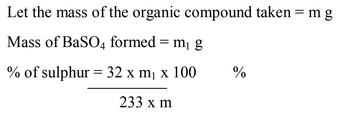## Phosphorous:

A known mass of an organic compound is heated with fuming nitric acid whereupon phosphorus present in the compound is oxidised to phosphoric acid. It is precipitated as ammonium phosphomolybdate, (NH4)3 PO4.12MoO3, by adding ammonia and ammonium molybdate.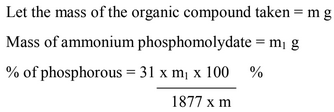## Oxygen:

The percentage of oxygen in an organic compound is usually found by difference between the total percentage composition (100) and the sum of the percentages of all other elements.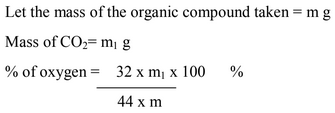How useful was this post?

Click on a star to rate it!

Average rating 5 / 5. Vote count: 1

No votes so far! Be the first to rate this post.

As you found this post useful...

Follow us on social media!

We are sorry that this post was not useful for you!

Let us improve this post!

Tell us how we can improve this post? Please mention your Email so that we can contact you for better feedback.